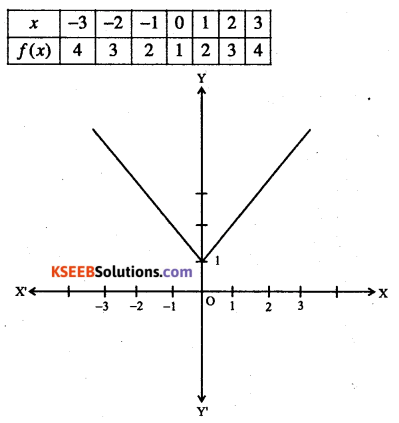# 1st PUC Maths Question Bank Chapter 2 Relations and Functions

Students can Download Maths Chapter 2 Relations and Functions Questions and Answers, Notes Pdf, 1st PUC Maths Question Bank with Answers helps you to revise the complete Karnataka State Board Syllabus and score more marks in your examinations.

## Karnataka 1st PUC Maths Question Bank Chapter 2 Relations and Functions

Question 1.
Define ordered pair.
Two numbers a and b listed in specific order and enclosed in parentheses form is called an ordered pair (a, b).
Keen Eye: Equality of two ordered pairs:
We have {a, b)-(c,d)⇔a-c and b – d.

Question 2.
Define a Cartesian product of two sets.
Let A and B two non-empty sets. Then, the Cartesian product of A and B is the set denoted by Ax B, consisting of all ordered pairs (a, b) such that a e A and be B.
A x B= {(a, b): a ∈ A, b∈B}
Keen Eye:

• If n(A) = p and n(B) = q, then n (A x B) = pq and n (B x A) = pq
• If at least one of A and B is infinite then AxB is infinite and B x A is infinite,
• In general, A x B ≠ B x A
• A x A x A = {(a, b, c) : a, b, c ∈A}. Here (a, b, c) is called an ordered triplet.Question 3.
If (x + 1, y – 2) = (3,1), find the values of x
Given (x + 1, y – 2) = (3,1)
⇒ x+1=3  ∴x=2
y-2=1  ∴ y=3

Question 4.
If $$\left(\frac{x}{3}+1, y-\frac{2}{3}\right)=\left(\frac{5}{3}, \frac{1}{3}\right)$$
Given $$\left(\frac{x}{3}+1, y-\frac{2}{3}\right)=\left(\frac{5}{3}, \frac{1}{3}\right)$$Question 5.
If P={a,b,c}and Q={r},from P×Q and Q x P.Are these two products equal?
PxQ = {(a,r),(b,r)(c,r)}
QxP = {(r, a), (r, b), (r, c)}
Clearly PxQ≠QxP

Question 6.
If the set A has 3 elements and the set B = {3, 4, 5}, then find the number of elements in
Given n(A) = 3; n(B) = 3.
∴ n(AxB) = 3×3 = 9

Question 7.
If G=(7, 8} and H={5, 4, 2), find G x II and
GxH = {(7,5),(7,4),(7,2),(8,5),(8,4),(8,2)}
HxG = {(5,7),(5,8),(4,7),(4,8),(2,7),(2,8)}

Question 8.
State whether each of the following statements are true or false. If the statement is false, rewrite the given statement correctly.
(i) If P={m, n} and Q = {n, m}, then P x Q = {(m, n), (n, m)}.
(ii) If A and B are non-empty sets, then A x B is a non-empty set of ordered pairs (at, y) such that x∈B and y∈A
(iii) If A = {1,2}, B = {3,4}, then A x {B∩φ ) = φ
(i) Given statement is false:
Correct statement:
PxQ={(m, n), (m, m), (n, n), (n, m)}.

(ii) Given statement is false:
Correct statement:
AxB = {(x, y) :x∈A, y ∈B}.                                 ‘

(iii) True statement,

Question 9.
If A x B = {(a, x), (a, y), (b, x), (b, y)}. Find A and
A = {a, b} and B – {x, y}

Question 10.
If A x B = {(p, q), (p, r), (m, q), (m, r)}, find A and
A = set of first elements = {p, m}
B = set of second elements = {q, r}Question 11.
Let A = (1, 2}, B = [1, 2, 3, 4}, C = { 5, 6} and D = (5,6,7,8}. Verify that
(i) A x (B∩C) = (A x B)∩(A x C).
(ii) A x C is a subset
(i) B∩C = { }
∴ Ax(B∩C)=φ ………….. (1)
A x B = {(1, 1), (1, 2), (1, 3), (1, 4), (2, 1), (2, 2), (2, 3), (2,4)}
A x C = {(1, 5), (1,6), (2, 5) (2,6)}
∴ (A x B) ∩ (A x C) = φ ………………. (2)
From (1) and (2), we get
A x (B∩C) = (A x B)∩(A x C)

(ii) A x C = {(1, 5), (1,6), (2, 5), (2, 6)}
B x D = {(1, 5), (1, 6), (1, 7), (1, 8), (2, 5), (2, 6), (2, 7), (2, 8), (3, 5), (3, 6), (3, 7), (3, 8), (4, 5), (4, 6), (4, 7), (4, 8)}.
Clearly every elements of A x C is an element of B x D.
A x C ⊂B x D.

Question 12.
Let A = {1, 2, 3}, B = {3, 4} and C = {4, 5, 6}. Find
(i) A x (B ∩ C)
(ii) (A x B) ∩ (A x C)
(iii) A x (B∪C)
(iv) (A x B)∪(A x C)
(i) B∩C={4}
A x (B∩C) = (1,4), (2, 4), (3,4)}

(ii) A x B = {(1, 3), (1,4), (2, 3), (2, 4), (3, 3), (3, 4)}
A x C = {(1, 4), (1, 5) (1, 6), (2, 4), (2, 5), (2, 6), (3, 4), (3, 5), (3, 6)}
(A x B)∩(A x C)= {(1, 4), (2, 4), (3, 4)}

(iii) B ∪ C={3,4, 5, 6}
∴ Ax(B∪C)  = {(1, 3), (1, 4), (1, 5), (1, 6), (2, 3), (2, 4), (2, 5), (2, 6), (3, 3), (3, 4), (3, 5), (3, 6)}

(iv) A x B = {(1, 3), (1, 4), (2, 3), (2, 4), (3, 3), (3, 4)}
A x C = {(1, 4), (1, 5), (1, 6), (2, 4), (2, 5), (2, 6), (3, 4), (3, 5), (3, 6)}
(A x B)∪(A x C) = {(1, 3), (1, 4), (1, 5), (1, 6), (2, 3), (2, 4), (2, 5), (2, 6) (3, 3), (3, 4), (3, 5), (3,6)}.

Question 13.
Let A = {1, 2} and B = {3, 4}. Write A x B. How many subsets will A x B have? List them.
Given A = {1, 2} and B = {3,4}
A x B= {(1, 3), (1,4), (2, 3), (2, 4)}
∴n (A x B) = 4
Number of subsets of A x B = 24=16
Subsets of A x B are: A x B, φ, {(1, 3)}, {(1, 4)}, {(2, 3)}, {(2, 4)}, {(1, 3), (1, 4)}, {(1, 3), (2, 3)}, {(1, 3), (2,4)}, {(1,4), (2, 3)}, {(1, 4), (2, 4)} {(2, 3), (2, 4)}, {(1, 3), (1, 4), (2, 3)}, {(1, 3), (1, 4), (2, 4)}, {(1,4), (2, 3), (2, 4)}, {(2, 3), (2,4), (1, 3)}.

Question 14.
Let A and B be two sets such that n(A) = 3 and n(B) = 2. If (x, 1), (y, 2), (z, 1) are in A x B, find A and B, where x, y, z are distinct elements.
A = {x, y, z} and B = {1, 2}.

Question 15.
The Cartesian product A x A has 9 elements along which are found (-1, 0) and (0,1). Find the set A and the remaining elements Ax A.
Given n(A x A) = 9 = 32
⇒n(A) = 3
But (-1, 0) and (0, 1) are in A x A
∴ A= {-1,0,1}.
Remaining elements of A x A: (-1, -1), (-1, 1),
(0,-1), (0,0), (1,-1), (1,0), (1,1).

Question 16.
If P = {1,2}, form the set
P x P x P = {(1, 1, 1), (1, 1, 2), (1, 2, 1),
(1, 2,2), (2, 1,1), (2, 1, 2), (2, 2,1), (2, 2, 2)}

Question 17.
If A = {-1,1}, find A x A x A.
A x A x A = {(-1, -1, -1), (-1, -I, 1), (-1, 1, -1), (-1, 1, 1), (1, -1, -1), (1, -1, 1), (1,1,-1), (1,1,1)}

Question 18.
If R is the set of all real numbers, what do the Cartesian products R x R and R x R x R represent?
We have R x R = {(x, y) : x, y ∈ R } which represents the coordinates of all the points in two dimensional space and R x R x R = {(x, y, z)  x,y,z ∈ R } which represents the coordinates of all the points in three-dimensional space.

Question 19.
Define a relation.
A relation R from a non-empty set A to non empty set B is a subset of the Cartesian product A x B.Question 20.
Define domain of a relation.
The set of all first elements of the ordered pairs in a relation R from a set A to a set B is called the domain of the relation R.

Question 21.
Define range of a relation.
The set of all second elements in a relation R from a set A to a set B is called the range of the relation R. The whole set B is called the co-domain of the relation R.

Question 22.
Let A = {1, 2, 3, 4, 5, 6}. Define a relation R from A to A by R = {(x,y): y = x + 1}
(i) Depict this relation using an arrow diagram
(ii) Write down the domain, condomain and range of
Given R = {(x, y): y = x + 1}
= {(1, 2), (2, 3), (3, 4), (4, 5), (5, 6)}Domain = {1, 2, 3,4, 5,}; Co-domain = A
Range = {2, 3,4, 5, 6}.

Question 23.
Let A = {1, 2, 3, ………….14}. Define a relation R from A to A by R = {(x, y): 3x – y = 0, x,
y ∈ A}. Write down its domain, co-domain and range.
Given R = {(x, y): 3x -y = 0, x, y ∈ A}
= {(1, 3), (2, 6), (3, 9), (4,12)}
Domain = {1, 2, 3,4}
Co-domain = A Range = {3, 6, 9,12}

Question 24.
Define a relation R on the set N of natural numbers by R – {(x, y) : y = x + 5, x is a natural number less than 4; x, y ∈ . N}. Depict this relationship using roster form. Write down the domain and the range.
Given R = {(x, y): x, y ∈ N and y = x + 5, x < 4}
= {(1,6), (2,7), (3, 8)}
Domain = {1, 2, 3}
Range = {6, 7, 8}Question 25.
A = {1, 2, 3, 5} and B = (4, 6, 9}. Define a relation R from A to B by R = {(x, y): the difference between x and y is odd; x ∈ A, y∈ B}. Write R in roster form.
Given A = {1, 2, 3, 5} and B = {4, 6, 9}
R = {(x, y): the difference between x and y is odd; x∈ A,y∈B}
= {(1,4), (1, 6), (2, 9), (3, 4), (3, 6), (5,4), (5, 6)}

Question 26.
The figure shows a relationship between the sets P and Write this relation
(i) in set- builder-form
(ii) roster form. What is its domain and range?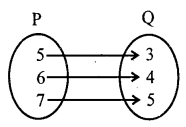Given P={5,6,7} and Q={3,4,5}
(i) Set builder form
R= {(x,y):x-y = 2; x∈P,y ∈Q)

(ii) Roster form
R = {(5, 3), (6,4), (7, 5)}
Domain of R = P
Range of R = Q.

Question 27.
Let A = {1, 2, 3, 4, 6}. Let R be the relation on A defined by {(a, b): a, b ∈A, b is exactly divisible by a},
(i) Write R in roster form
(ii) Find the domain of R
(iii) Find the range of R
Given A = { 1, 2, 3,4, 6}
R- {(a, b),a,b∈A,bis exactly divisible by a}

(i) Roster form:
R = {(1, 1), (1, 2), (1, 3), (1, 4), (1, 6), (2, 2), (2, 4), (2, 6), (3, 3), (3, 6), (4,4), (6, 6)}

(ii) Domain of R = {1, 2, 3,4, 6} = A

(iii) Range of R = {1, 2, 3,4, 6} = AQuestion 28.
Determine the domain and range of the relation R .defined by R = {(x, x + 5): x e {0,1, 2,3,4,5}}.
Given R = {(0, 5), (1, 6), (2, 7), (3, 8), (4, 9), (5, 10)}.
Domain of R = {0, 1, 2, 3,4, 5}
Range of R = {5, 6, 7, 8, 9, 10}

Question 29.
Write the relation R = {(x, x3) : x is a prime number less than 10} in roster form.
Given R = {(x, x3): x g {2, 3, 5, 7}}
= {(2, 8), (3, 27), (5, 125), (7, 343)}

Question 30.
Let A – {x, y, z} and B = (1, 2}. Find the number of relations from A to
Given n(A) = 3 and n(B) = 2
∴ n (A x B) = 3 x 2 = 6
Number of relations from A to B = 2n (A x B) = 26 = 64

Question 31.
Let R be the relation on Z defined by R = {(a, b): a, b ∈ Z, a – b is an integer}. Find the domain and range of
Given: R = {(a, b): a, b ∈Z,a-b is an integer} Domain of R-Z Range of R = Z

Question 32.
Let R be a relation from Q to Q defined by R = {(a, b)\ a,b ∈Q and a – b ∈ Z}. Show that (a, a) g R, for all a ∈Q
(ii) (a, b) ∈ R implies that (b, a) ∈R
(iii) (a, b) ∈ R and (b, c) ∈R implies that (a, c) ∈ R.
Given R = {{a, b): a,b∈Q and a-b ∈ Z)
(i) ∀ a ∈ Q, a – a = 0 ∈ Z ⇒ (a, a)∈R

(ii) Let (a, b) ∈ R⇒ a- b ∈ Z
b – a ∈ Z ⇒ (b, a) ∈ R

(iii) Let (a, b) and (b, c) g R ⇒ a – b ∈Z and
b – c ∈ Z
a- c = (a-b) + (b – c) ∈Z
∴ (a, c)∈ R

Question 33.
Let R be a relation from A to A defined by R = {(a, b): a,b ∈ N and a = b2} Are the following true?
(i) (a, a) ∈ R for all a ∈ A
(ii) (a, b) ∈ R, implies (b, a) ∈ R
(iii) (a, b) ∈ R, (b, c) ∈ R, implies (a, c) ∈ R.
Given R= {(a, b): a,b∈N and a = b2}
= {(1,1), (2,4), (3, 9), (4, 16),…}
(i) (a, a)∈ R for all a ∈ N is not true because (2, 2) ∉ R.
(ii) (a, b) ∈ R implies (b, a) ∈ R is not true, because  (2,4) ∈ R but (4,2) ∉ R
(iii) (a, b) ∈ R, (b, c) ∈ R implies (a,c) ∈ R is not true because (2,4) and (4,16) ∈ R but (2,16) ∉ R.Question 34.
Define a function.
A relation/from a set A to a set B is said to be a function if every element of set A has one and only one image in set B, and
we write f : A → B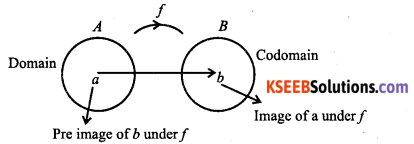Question 35.
Define
(i) a real valued function
(ii) a real function
A function which has either R or one of its subsets as its range is called a real valued function. A function f: A → B is said to be a real function if both A and B are subsets of R.

Question 36.
Let N be the set of natural numbers and the relation R be defined on N such that
R = {(x,y):y = 2x,x,y ∈ N} What is the domain, co-domain and range of R? Is this relation a function?
Given R = {x, y): y = 2x; x, y ∈ N}
Domain of R = set of natural numbers Co-domain of
R = set of natural number
Range of R = set of even natural numbers
Clearly, every natural number is related to unique image, so this relation is a function.

Question 37.
Examine each of the following relations given below and state in each case, giving reasons whether it is a function or not?
(i) R = {(2,1), (3,1), (4,2)}
(ii) R = {(2,2), (2,4), (3,3), (4,4)}
(iii) R = {(1, 2), (2, 3), (3, 4), (4, 5), (5, 6), (6,7)}
(i) Given R= {(2,1), (3,1), (4, 2)}
Here every element of domain is related to unique element of co-domain, so it is a function.

(ii) Given R = {(2, 2), (2,4), (3, 3), (4, 4)}
Since the element 2 has two images namely 2 and 4, so it is not a function.

(iii) Given R = {(1, 2), (2, 3), (3, 4), (4, 5), (5, 6), (6, 7)}
Since every element of domain is related to unique

Question 38.
Let N be the set of natural numbers. Define a real valued function f: N → N by
f(x) = 2x + 1. Using this definition, complete the table given below:

 X 1 2 3 4 5 6 7 y F : (1) = F : ( 2) = F : (3) = F : (4) = F : (5) = F : (6) = F : (7) =

Given function is f(x) = 2x + 1.

 X 1 2 3 4 5 6 7 y F:(1)=3 F:(2)=5 F:(3)=7 F:(4)=9 F:(5)=11 F:(6)=13 F:(7)=15

Question 39.
Which of the following relations are functions? Give reasons. If it is a function, determine its domain and range.
(i) {(2,1), (5,1), (8,1), (11,1), (14,1), (17,1)}
(ii) {(2, 1), (4, 2), (6, 3), (8, 4), (10, 5), (12, 6), (14,7)}
(iii) {(1,3), (1,5), (2,5)}.
(i) Clearly, every element of domain is related to unique element of co-domain, so it is a function.
Domain = {2, 5, 8, 11, 14, 17}
Range = {1}

(ii) Clearly, every element of domain is related to unique element of co-domain, so it is a function.
Domain = {2,4, 6, 8,10,12,14}
Range = {1,2, 3,4, 5, 6,7}

(iii) 1 is related to two elements of co-domain, namely 3 and 5, so it is not a function.Question 40.
Let A={1,2,3,4), B={1,5,9,11,15,16} and f = {(1,5),(2,9), (3,1), (4,5),(2, 11)). Are the
following true?
(i) f is a relation from A to B
(ii) f is a function from A to B. Justify your answer in each case.
(i) Every element off is an element of A x B, so f is a relation.
(ii) ‘f’ is not a function the element 2 has two images.

Question 41.
Let f be the subset of Z x Z defined by f ={(ab,a +b):a,b ∈z) Is f a function from Z to Z? Justify your answer.
Given f={(ab,a+b):a,b∈Z)
If a = 1 and b = 4 = ab = 4 and a+b=5
∴ (4,5) ∈ fIf a=2 and b=2⇒ab=4 and a+b=4
∴ (4,4) ∈ f∴ The element 4 has two images, so f is not a function.

Question 42.
The relation f is defined by
$$f(x)=\left\{\begin{array}{ll}{x^{2},} & {0 \leq x \leq 3} \\ {3 x,} & {3 \leq x \leq 10}\end{array}\right.$$
The relation g is defined by
$$g(x)=\left\{\begin{array}{ll}{x^{2},} & {0 \leq x \leq 2} \\ {3 x,} & {2 \leq x \leq 10}\end{array}\right.$$ .
Show that/is a function and g is not a function.
Since f(x) is unique for 0 ≤ x ≤ 10.
f(x) is a function. g(2) = 22 =4 and g(2) = 3(2) = 6
∴ z has two images under g.
∴ g is not a function.

Question 43.
Let f= {(1,1),(2,3),(0,-1),(-1,-3)} be a linear function from Z into Z. Find f(x) ).
Since/is a linear function.
∴f{x) = ax + b
But (0,-1) ∈ f . f(0) ∴ a(0) + b ⇒ -1 = b
Similarly, (1,1) ∈ f ∴ f(1) = a(1) +a(1)+b
⇒ 1=a+b ∴ a=2 ∴ f(x) = 2x -1

Question 44.
A function f is defined by f(x) = 2x-5. Write down the values of (i) f(0) (ii) f(17) (iii) f(-3).
Given: f(x) = 2x-5

• f(0) = 2(0) – 5 = -5
• f(17) = 2(17)-5 = 29
• f(-3) = 2(-3) – 5 = -11

Question 45.
The function ‘t’ which maps temperature in degree Celsius into temperature in degree. Fahrenheit is defined by $$t(c)=\frac{9 c}{5}+32$$. Find
(i) t (0)
(ii) t (28)
(iii) t (-10)
(iv) The value of c, when t(c) – 212Question 46.
$$\text { If } f(x)=x^{2}, \text { find } \frac{f(1 \cdot 1)-f(1)}{1 \cdot 1-1}$$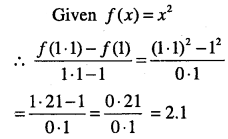Question 47.
Find the range of each of the following functions:
(i) f{x) = 2-3x, x∈R,x>0
(ii) f(x) = x2 + 2, x is a real number
(iii) f(x) = x, x is a real number.
(i) Given f(x) = 2-3x, x∈R,x>0
For x > 0,f(x) = 2 – 3x < 2
∴ Range of f= (-∞, 2)

(ii) Given f(x) = x2 + 2, x ∈ R
For x ∈ R, f(x) = x2 + 2 ≥ 2
Range of f = [2, ∞)

(iii) Given f(x) = x, x∈ R For r∈ E, f(x) = x∈R
Range of f = RQuestion 48.
Let A = (9, 10, 11, 12, 13} and let f:A→N be defined by f(n) = the highest prime factor of n. Find the range of f.
Given f(n) = the highest prime factor of n.
f(9) = the highest prime factor of 9 = 3
f(10) = the highest prime factor of 10 = 5
f(11) = the highest prime factor of 11 = 11
f(12) = the highest prime factor of 12 = 3
f(13) = the highest prime factor of 13 = 13
Range of f ={3,5,11,13}

Question 49.
$$\text { Let } f=\left\{\left(x, \frac{x^{2}}{1+x^{2}}\right): x \in \mathbb{R}\right\}$$ be a function from R to R .Determine the range of f.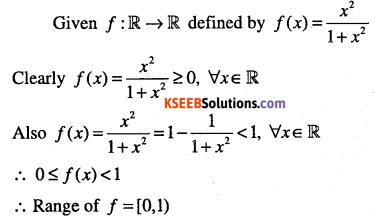Question 50.
Find the domain of the function $$f(x)=\frac{x^{2}+3 x+5}{x^{2}-5 x+4}$$
Given $$f(x)=\frac{x^{2}+3 x+5}{x^{2}-5 x+4}$$
f(x) is defined for all real numbers except x2 – 5x + 4 = 0
But x2 -5x + 4 = (x-4) (x-1)
Domain of f= R-{1,4}

Question 51.
Find the domain of the function
$$f(x)=\frac{x^{2}+2 x+1}{x^{2}-8 x+12}$$
$$f(x)=\frac{x^{2}+2 x+1}{x^{2}-8 x+12}$$
is not defined when
x2 – 8+12 = 0.
∴ (x-6)(x-2) = 0
∴ Domain of f = R-{2,6}

Question 52.
Find the domain and range of the following real functions:
(i) $$f(x)=-|x|$$
(ii) $$f(x)=\sqrt{9-x^{2}}$$
(iii) $$f(x)=\sqrt{x-1}$$
(iv) $$f(x)=|x-1|$$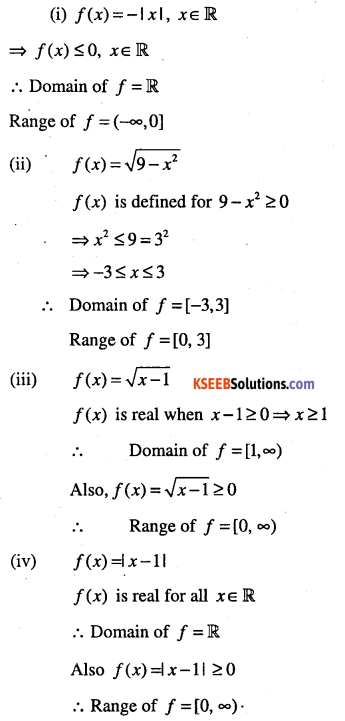Remark: Operations of functions:
(i) Addition of two real functions:
Let f: X → R and g : X → R Then.
(f + g):X → R; (f + g)(x) = f(x) + g(x) for all x ∈ X

(ii) Difference of two real functions:
Let f : X → R and g : X → R Then.
(f – g): X → R; (f- g)(x) = f(x) – g(x) for all x ∈ X

(iii) Scalar multiplication of a function:
Let f: X → R and let ‘a’ he a scalar. Then,
(αf):X → R; (fg)(x) = f(x) for all x ∈ X

(iv) Multiplication of two real functions:
Let f: X → R and g : X → R .Then
(fg): X → R; (fg)(x)= f(x)g(x), for all x ∈ X

(v) Quotient of two real functions:
Let f: X → R and  g : X → R for all x for which g(x) ≠ 0. Then
$$\left(\frac{f}{g}\right): X \rightarrow \mathbb{R} ;\left(\frac{f}{g}\right)(x)=\frac{f(x)}{g(x)}$$Question 53.
Let f(x) = x2 and g(x) = 2x + 1 be two real functions. Find (f+g)(x),(f-g)(x)
$$(f g)(x),\left(\frac{f}{g}\right)(x)$$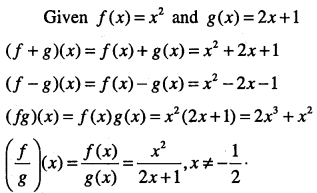Question 54.
Let $$f(x)=\sqrt{x} \text { and } g(x)=x$$ be two functions defined over the set of non-negative real numbers. Find (f+ g)(x), (f – g)(x) $$(f g)(x) \text { and }\left(\frac{f}{g}\right)(x)$$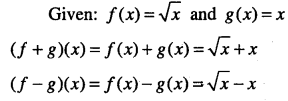Question 55.
Let f,g: R→R be defined, respectively by f(x) = x + 1,g(x) = 2x-3- Find f + g,f-g and $$\frac{f}{g}$$
Given: f(x) = x + 1,g(x) = 2x-3
(f + g)(x) = f (x) + g(x) = x + 1 + 2x-3 = 3x-2
(f – g)(x) = fix) – g(x) = x + 1-2x + 3 = -x + 4
$$\left(\frac{f}{g}\right)(x)=\frac{f(x)}{g(x)}=\frac{x+1}{2 x-3}, x \neq \frac{3}{2}$$

Question 56.
Define an identity function and draw its graph also find its domain and range.
The function f: M → R; f(x) = x for a II x∈R is called an identity function on R.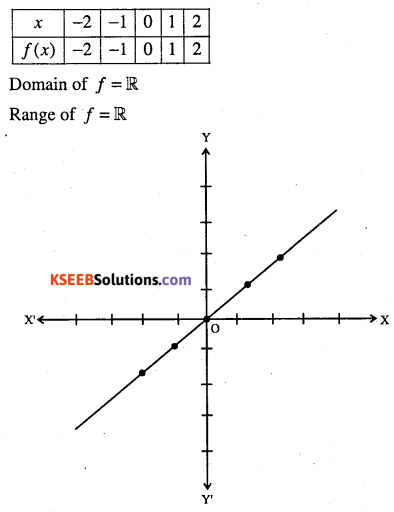Question 57.
Define a constant function and draw its graph also find its domain and range.
Let c be a fixed real number. Then, the function f : R→R, f(x) = c for all x∈R is called the constant function.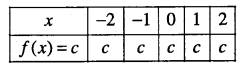f(x) is defined for all real number,
∴ Domain = M
Range = { c }Question 58.
Define a polynomial function.
A function f : M → R is said to be polynomial function if for each x in IR,
y = f (x) = a0 + axx + a2x2 + ……………. + anxn
where n is a non-negative integer and a0,a1,a2………………….an ∈ R .

Question 59.
Draw the graph of the function f (x) = x2 and write its domain and range.
Given function: f(x)= x2Domain = R
Range = set of non-negative reals.Question 60.
Draw the graph of the function f: R → R defined by f(x) = x3 Find its domain and range.
Let f : R → R: f(x) = x3, ∀ ∈ R
Then, domain of f = R and range of f = R . we have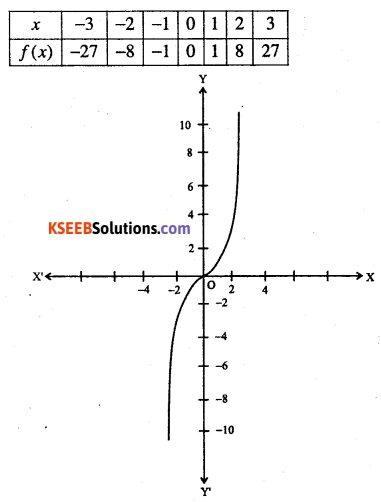Question 61.
Define a rational function
The functions of the type $$\frac{f(x)}{g(x)}$$ where f(x) g(x) and g(x) are polynomial functions of x, defined in a domain, where g(x) ≠ 0

Question 62.
Let f : R – {0} → R defined by $$f(x)=\frac{1}{x}, \forall x \in \mathbb{R}-\{0\}$$ . Find its domain and x range. Also, draw its graph.
Given function is f : R – {0} → R defined by $$f(x)=\frac{1}{x}$$
∴ Domain = R-{0} and range =R-{0}

 X -4 -2 -1 -0-5 -0-25 0-25 0-5 1 2 f(x)=1/x -0-25 -0-5 -1 -2 -4 4 2 1 0-5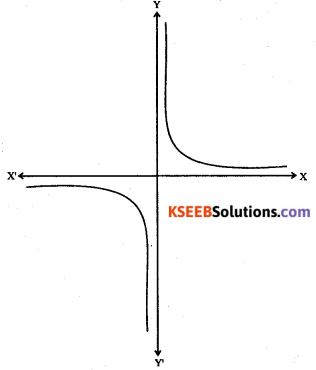Question 63.
Define a modulus function. Find its domain and range. Also, draw its graph.
Let f: R → R defined by f(x) =1 x I, for each x ∈ R, is called modulus function.
$$\text { i.e., } f(x)=|x|=\left\{\begin{array}{ll}{x,} & {\text { if } x \geq 0} \\ {-x,} & {\text { if } x<0}\end{array}\right.$$Domain = R
Range = set of non negative real numbers

Question 64.
Define Signum function. Draw its graph and find its domain and range.
The function f: R → R defined by
$$f(x)=\left\{\begin{array}{lll}{1,} & {\text { if }} & {x>0} \\ {0,} & {\text { if }} & {x=0} \\ {-1,} & {\text { if }} & {x<0}\end{array}\right.$$ is called signum function
We haveDomain = R
Range = {-1,0,1}

Question 65.
Define a greatest integer function. Draw its graph and find its domain and range.The function f : R → R define by f(x) = [x], x∈ R assumes the value of the greatest integer, less than or equal to x. Such a’ function is called the greatest integer function or step function. We have
[x] = -2 for – 2 ≤ x < -1
[x] = -1 for -1≤x<0
[x] = 0 for 0≤x≤1
[x] = 1 for 1 ≤ x < 2
[x] = 2 for 2 ≤ x < 3.
Hence, domain of f = R and range = Z.

Question 66.
Define a linear function.
The function f : R → R defined by f(x) = mx + c, x ∈ R is called linear function, where m and c are constant.Question 67.
Let R be the set of real numbers. Define the real function f : R →R by f(x) = x + 10 and sketch the graph of this function.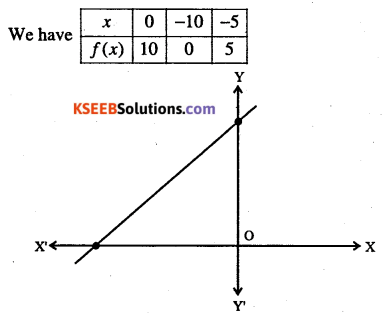$$f(x)=\left\{\begin{array}{cl}{1-x,} & {x<0} \\ {1,} & {x=0} \\ {x+1,} & {x>0}\end{array}\right.$$.